# 泛化理论整理

07/30 11:24

ERM模型empirical risk minimization

• 广泛数据分布(Population distribution)
1. 特征Feature(x∈X⊂$R^d$)：比如一张图片
2. 标签Label(y∈Y⊂R)：比如猫狗分类

• 训练数据集(Trainning Dataset)

• 最小化的经验损失(empirical risk minimization)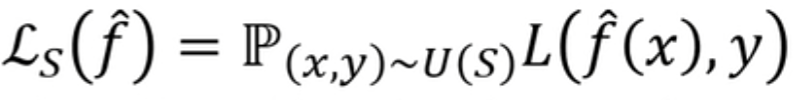• 模型$y=f_θ(x)$

• 推理(Evaluation)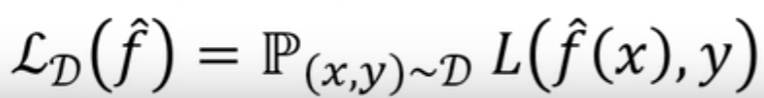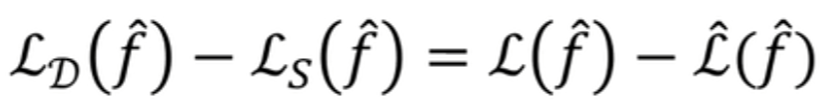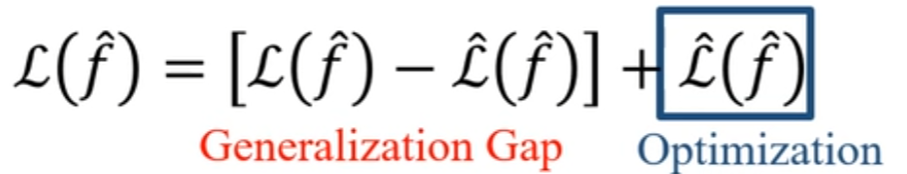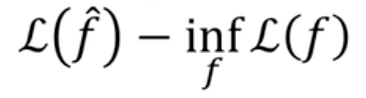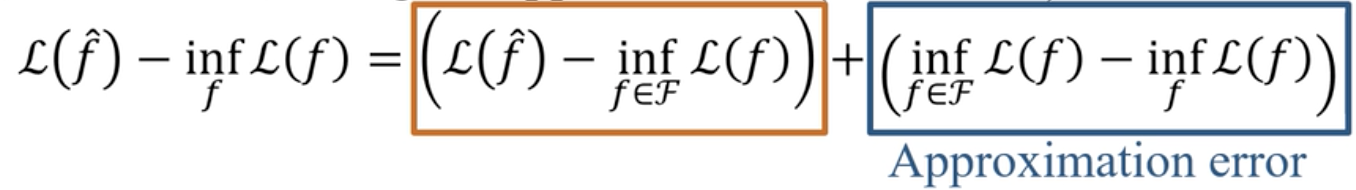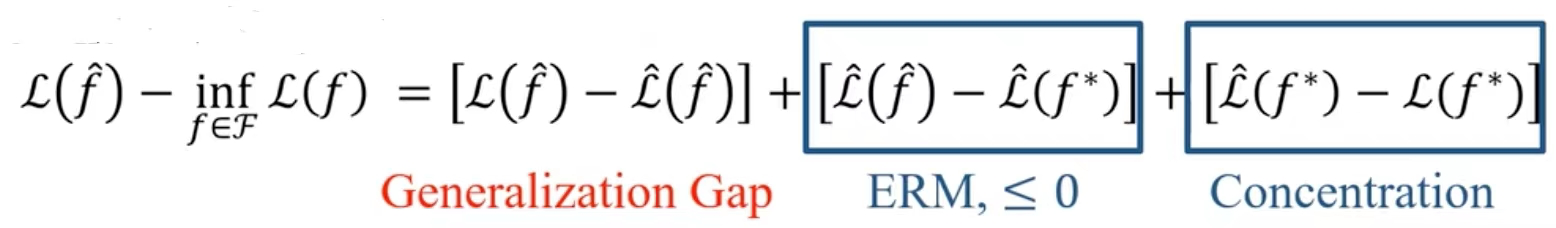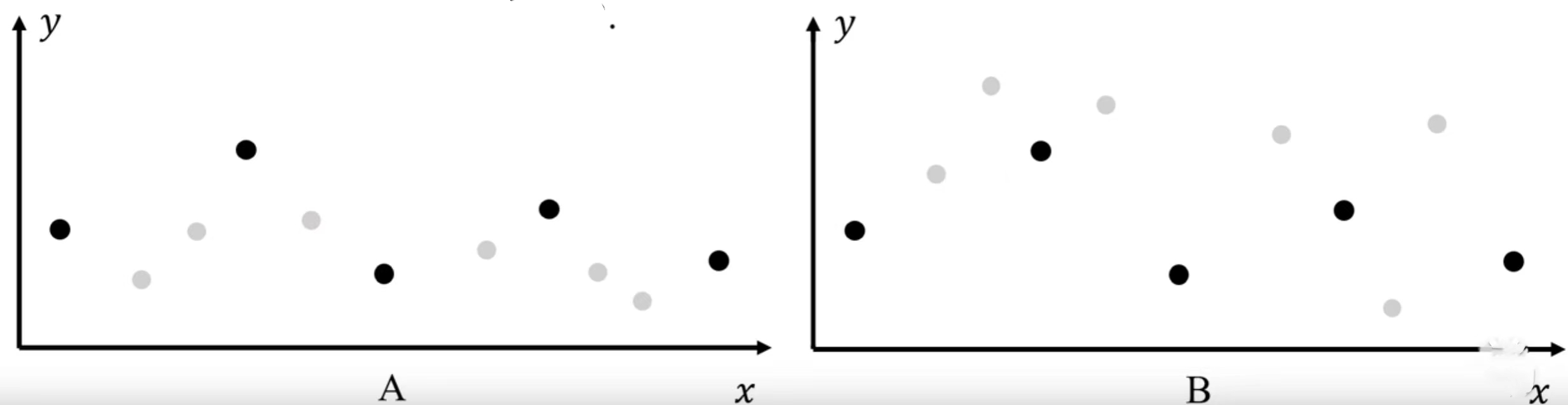1. 存在着一个模型f:X->{0,1}使得损失函数$L_D(f)=0$，即存在着一个好的预测模型。
2. 对于训练样本的分布S，类比于全样本分布D，仅仅只有$1\over 7$的概率能使得广泛损失$L_D(A(S))≥{1\over 8}$，即我们无法找到1中的好模型。

PAC(Probably Approximately Correct) Learning

PAC理论的前提是需要知道一些先验知识的。

1. Probably(概率)：对于任意的分布只要满足先验。
2. Approximately(近似)：抽取出来的训练集不是太差。
3. Correct(正确)：就能够找到一个好的算法找到这个最好的模型

$m_H(ɛ,δ)≤[{log(|H|/δ)\over ɛ}]$0 评论
0 收藏
0